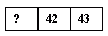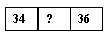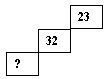Email us to get an instant 20% discount on highly effective K-12 Math & English kwizNET Programs!

#### Online Quiz (WorksheetABCD)

Questions Per Quiz = 2 4 6 8 10

### Grade 1 - Mathematics3.19 Puzzle Pictures• The difference between any two numbers in any column is 10.
• The difference between any two numbers in any row is 1.
• The difference between any two numbers in any diagonal columns is 9.
Example:
Using the Hundred Table, find the missing number.
 13 14 ___
13 14 __ are numbers lying in a row.

Example:
Using the Hundred Table, find the missing number.
 23 __ 43
These are examples of numbers lying in a column.

Example:
Using the Hundred Table, find the missing number.
 10 19 ?
10 19 ? are examples of numbers lying diagonally.

Directions: Answer the following questions. Also write at least ten examples of your own.
 Q 1: Using the Hundred Table, find the missing number in the figure.Answer: Q 2: Using the Hundred Table, find the missing number in the figure.Answer: Q 3: Using the Hundred Table, find the missing number in the figure.Answer: Q 4: Using the Hundred Table, find the missing number in the figure.Answer: Q 5: Using the Hundred Table, find the missing number in the figure.Answer: Q 6: Using the Hundred Table, find the missing number in the figure.Answer: Q 7: Using the Hundred Table, find the missing number in the figure.Answer: Q 8: Using the Hundred Table, find the missing number in the figure.Answer: Question 9: This question is available to subscribers only! Question 10: This question is available to subscribers only!